## ABSTRACT

This paper presents part of the work done within the project ‘Advanced Numerical Simulation and Performance Evaluation of WAM-V ® in Spray Generating Conditions’ developed by the International Center for Numerical Methods in Engineering (CIMNE) under Navy Grant N62909-12-1-7101 issued by the Office of Naval Research Global.

One of the primary goals of that project was the development of a computational model for simulation of the Wave Adaptive Modular Vessel (WAM-V®) under spray generating conditions.

For this purpose, a Semi-Lagrangian Particle Finite Element Method (SL-PFEM) has been applied. This is the latest development within the framework of the so-called Particle Finite Element Method (PFEM), using the X-IVAS (eXplicit Integration along the Velocity and Acceleration Streamlines) scheme.

In this paper we demonstrate the applicability of the SL-PFEM using the X-IVAS scheme for the simulation of the Wave Adaptive Modular Vehicle under spray generating conditions.

## INTRODUCTION

A Wave Adaptive Modular Vessel (WAM-V®) is a new class of ship that uses inflatable flexible hulls to conform to the surface of the water. It is similar in design to a catamaran, in that it has a twin hull design and no keel. However, the superstructure is not rigidly attached to the hulls; it uses shock absorbers and ball joints to articulate the vessel, which allows WAM-V to conform to the surface of the water while mitigating the stresses transmitted to the structure. Moreover, the inflatable hulls help to absorb the high frequency wave-loads. These features allow WAM-V to travel efficiently with low wave resistance in rough seas, by surfing on top of the waves rather than cut through them.

The objective of the WAM-V is to be a lightweight watercraft capable of moving fast and efficiently on the surface of the sea. WAM-Vs are designed to allow for a variety of applications for either manned or unmanned operations and can be built in different lengths to match specific services.

This paper presents part of the work done in the project ‘Advanced Numerical Simulation and Performance Evaluation of WAM-V ® in Spray Generating Conditions’ developed by the International Center for Numerical Methods in Engineering (CIMNE) under Navy Grant N62909-12-1-7101 issued by the Office of Naval Research Global. The scope of that project included the performance analysis of the WAM-V in waves, taking into account the flexibility of the ship hulls, using fluid-structure interaction computational models (see Figure 1). However, the focus of this paper is one of the primary concerns of that project; the development of a computational model for simulation of the WAM-V under spray generating conditions. In this regards, the final goal was to develop and demonstrate a computational engineering solver that could be used to design strategies to reduce the spray generation of the vessel.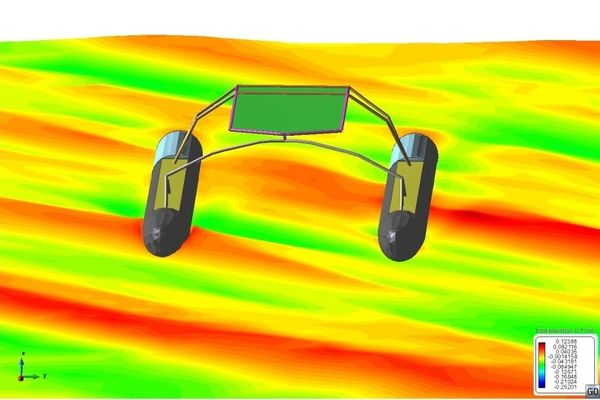Figure 1. Snapshot of a fluid-structure interaction analysis of the WAM-V in irregular sea (colormap shows free surface elevation).

When the rest of the components of drag are significantly reduced, the viscous components and any other source of energy dissipation induced by the movement of the vessel, become increasingly important. Because of the inflatable nature of the hulls of the WAM-V, there is little room for hydrodynamic shape optimization. Furthermore, as a consequence of the shape of the hulls, it is likely that they will generate spray when touching the sea surface. Therefore, spray might become an important source of energy dissipation in these little optimized hull shapes. In addition, excessive spray generation can increase the difficulties associated with operating the ship in certain cases (e.g. the possibility of the spray reaching the deck of the craft is a design issue depending on the particular operations the vessel is set to perform).

Therefore it becomes obvious the need to characterize and reduce the spray generation, in order to increase the range of operation of this class of vessels. Furthermore, there is a need to understand the dynamics of the vessel and the hulls in different sea states and the generation of spray when sailing in a seaway.

The Particle Finite Element Method (PFEM, Idelsohn et al., 2004) is a versatile framework for the analysis of fluid-structure interaction problems. The PFEM combines Lagrangian particle-based techniques with the advantage of the integral formulation of the Finite Element Method (FEM).

It has been shown (Idelsohn et al., 2004; Becker, 2015) to successfully simulate a wide variety of complex engineering problems, e.g. free-surface/multi-fluid flows with violent interface motions, multi-fluid mixing and buoyancy-driven segregation problems etc.

The latest development within the framework of the PFEM is the X-IVAS (eXplicit Integration along the Velocity and Acceleration Streamlines) scheme (Idelsohn et al., 2012). It is a semi-implicit scheme built over a Semi-Lagrangian (SL) formulation of the PFEM.

In this paper we present the application of the SL-PFEM using the X-IVAS scheme for the simulation of the Wave Adaptive Modular Vehicle under spray generating conditions.

## SEMI-LAGRANGIAN PARTICLE FINITE ELEMENT METHOD

Notation: Vectors are written using bold italic font and matrices are written using bold upright font.

The independent variables in Lagrangian kinematics are ${\textstyle \lbrace \lambda ,t\rbrace }$ , where ${\textstyle \lambda }$ represents a label to identify particles and ${\textstyle t}$ represents the time elapsed after labeling.

The primary dependent variable is the fluid particle trajectory denoted as ${\textstyle {\mathit {\boldsymbol {X}}}\left(\lambda ,t\right)}$ . The independent variables in Eulerian kinematics are ${\textstyle \left({\mathit {\boldsymbol {x}}},t\right)}$ , where ${\textstyle {\mathit {\boldsymbol {x}}}}$ denotes the spatial coordinates. The primary dependent variable is the fluid velocity ${\textstyle {\mathit {\boldsymbol {u}}}\left({\mathit {\boldsymbol {x}}},t\right)}$ .

Consider the Eulerian description of the incompressible Navier-Stokes equations.

 ${\textstyle {\partial }_{t}{\mathit {\boldsymbol {u+}}}\left({\mathit {\boldsymbol {u}}}\cdot \nabla \right){\mathit {\boldsymbol {u-}}}\nu \Delta {\mathit {\boldsymbol {u}}}+\nabla (p/\rho )=}$f
(1)
 $\nabla \cdot {\mathit {\boldsymbol {u}}}=0$
(2)

where ${\textstyle \nu }$ is the kinematic viscosity and ${\textstyle p\left({\mathit {\boldsymbol {x}}},t\right)}$ , ${\textstyle {\mathit {\boldsymbol {f}}}\left({\mathit {\boldsymbol {x}}},t\right)}$ are the pressure and the external acceleration fields, respectively.

The effective acceleration field ${\textstyle {\mathit {\boldsymbol {a}}}\left({\mathit {\boldsymbol {x}}},t\right)\,}$ in the fluid domain is obtained from the momentum balance equation of the flow.

 ${\textstyle {\mathit {\boldsymbol {a}}}={\partial }_{t}{\mathit {\boldsymbol {u+}}}\left({\mathit {\boldsymbol {u}}}\cdot \nabla \right){\mathit {\boldsymbol {u}}}=}$$\nu \Delta {\mathit {\boldsymbol {u}}}-\nabla \left({\frac {p}{\rho }}\right)+$f
(3)

Note that the functional dependence on the independent variables is suppressed in equations (1), (2) and (3) for brevity.

The fundamental principle of kinematics relates the Eulerian description of the flow with the Lagrangian description as follows.

 ${\mathit {\boldsymbol {U}}}\left(\lambda ,t\right):={\frac {d{\mathit {\boldsymbol {X}}}\left(\lambda ,t\right)}{dt}}=$${\mathit {\boldsymbol {u}}}\left({\mathit {\boldsymbol {X}}}\left(\lambda ,t\right),t\right)$
(4)
 ${\frac {d{\mathit {\boldsymbol {U}}}\left(\lambda ,t\right)}{dt}}={\frac {{d}^{2}{\mathit {\boldsymbol {X}}}\left(\lambda ,t\right)}{d{t}^{2}}}=$${\mathit {\boldsymbol {a}}}\left({\mathit {\boldsymbol {X}}}\left(\lambda ,t\right),t\right)$
(5)

The basic idea of the X-IVAS scheme is to update the fluid particle position and velocity within a time-step ${\textstyle {t}^{n}\,\leq t\,\leq \,{t}^{n+1}}$ using

 ${\frac {d{\mathit {\boldsymbol {X}}}^{h}\left(\lambda ,t\right)}{dt}}={\mathit {\boldsymbol {u}}}^{h}\left({\mathit {\boldsymbol {X}}}^{\mathit {\boldsymbol {h}}}\left(\lambda ,t\right),{t}^{n}\right)=$${\boldsymbol {A}}{\mathit {\boldsymbol {\,}}}{\mathit {\boldsymbol {X}}}^{h}\left(\lambda ,t\right)+$${\mathit {\boldsymbol {b}}}$
(6)
 ${\frac {d{\mathit {\boldsymbol {U}}}^{h}\left(\lambda ,t\right)}{dt}}={\mathit {\boldsymbol {a}}}^{h}\left({\mathit {\boldsymbol {X}}}^{h}\left(\lambda ,t\right),{t}^{n}\right)=$${\boldsymbol {C}}{\mathit {\boldsymbol {\,}}}{\mathit {\boldsymbol {X}}}^{h}\left(\lambda ,t\right)+$${\mathit {\boldsymbol {d}}}$
(7)

where ${\textstyle {\mathit {\boldsymbol {u}}}^{h}\left({\mathit {\boldsymbol {x}}},t\right)}$ and ${\textstyle {\mathit {\boldsymbol {a}}}^{h}\left({\mathit {\boldsymbol {x}}},t\right)}$ denote spatially continuous piecewise linear approximations of the velocity and acceleration defined on a background simplicial mesh. The matrices ${\textstyle {\boldsymbol {A}}}$, ${\textstyle {\boldsymbol {C}}}$ and the vectors ${\textstyle {\mathit {\boldsymbol {b}}}}$, ${\textstyle {\mathit {\boldsymbol {d}}}}$ are spatially piecewise constant and depend on the time ${\textstyle {t}^{n}}$. The particle trajectory and its velocity computed in this manner are denoted as ${\textstyle {\mathit {\boldsymbol {X}}}^{\mathit {\boldsymbol {h}}}\left(\lambda ,t\right)}$ and ${\textstyle {\mathit {\boldsymbol {U}}}^{\mathit {\boldsymbol {h}}}\left(\lambda ,t\right)}$ , respectively.

Nielson and Jung (1999) presented formulas in 2D and 3D to compute the closed-form analytical solution of tangent curves for piecewise linear vector fields defined over simplicial meshes.

Thus, the Nielson--Jung formulas can be used to compute the analytical solution of (6). Idelsohn et al. (2012) presented a procedure to compute the analytical solution of (6) and (7) in 2D. However the Nielson--Jung formulas and the calculation procedure described by Idelsohn et al. to compute the analytical solution are not numerically stable; loss of significance occurs due to subtractive cancellations near removable singularities. Recently, Nadukandi (2015) presented numerically stable formulas in 2D and 3D for the closed-form analytical solution of (6) and (7).

In the following, we briefly describe the algorithm to implement the SL-PFEM using the X-IVAS scheme.

First the Lagrangian advection of the particles: ${\textstyle {\mathit {\boldsymbol {X}}}^{\mathit {\boldsymbol {h}}}\left(\lambda ,{t}^{n}\right)\,\,\rightarrow }$ ${\textstyle {\mathit {\boldsymbol {X}}}^{\mathit {\boldsymbol {h}}}\left(\lambda ,{t}^{n+1}\right)}$ and ${\textstyle {\mathit {\boldsymbol {U}}}^{\mathit {\boldsymbol {h}}}\left(\lambda ,{t}^{n}\right)\,}$ ${\textstyle \rightarrow \,{\mathit {\boldsymbol {U}}}^{\mathit {\boldsymbol {h}}}\left(\lambda ,{t}^{n+1}\right)}$ are done solving the following equations

 ${\mathit {\boldsymbol {X}}}^{h}\left(\lambda ,{t}^{n+1}\right)=={\mathit {\boldsymbol {X}}}^{h}\left(\lambda ,{t}^{n}\right)+$$\int _{{t}^{n}}^{{t}^{n+1}}{\mathit {\boldsymbol {u}}}^{h}\left({\mathit {\boldsymbol {X}}}^{h}\left(\lambda ,\tau \right),{t}^{n}\right)d\tau$
(8)
 ${\hat {\mathit {\boldsymbol {U}}}}^{h}\left(\lambda ,{t}^{n+1}\right)={\mathit {\boldsymbol {U}}}^{h}\left(\lambda ,{t}^{n}\right)$ $-\gamma \int _{{t}^{n}}^{{t}^{n+1}}\nabla \left({\frac {p\left({\mathit {\boldsymbol {X}}}^{h}\left(\lambda ,\tau \right),{t}^{n}\right)}{\rho }}\right)d\tau +$$\theta \int _{{t}^{n}}^{{t}^{n+1}}\left(\nu \Delta {\mathit {\boldsymbol {u}}}^{h}\left({\mathit {\boldsymbol {X}}}^{h}\left(\lambda ,\tau \right),{t}^{n}\right)+{\mathit {\boldsymbol {f}}}^{h}\left({\mathit {\boldsymbol {X}}}^{h}\left(\lambda ,\tau \right),{t}^{n}\right)\right)d\tau$
(9)

where ${\textstyle {\hat {\mathit {\boldsymbol {U}}}}^{h}\left(\lambda ,{t}^{n+1}\right)}$ is an estimate of the velocity of the particle, and ${\textstyle \,\gamma ,\theta \,\in \,\left[0,1\right]\,}$ depend on the integration scheme to be used.

Then the data advected with particles is projected onto a background finite element (FE) mesh. These data include the particle velocities and identities (in multi-fluid flows) among other problem dependent information. Then, the interface between multiple fluids is reconstructed (Becker, 2015) on the FE mesh using the advected particle identities. Appropriate enrichments are determined (Becker, 2015) for the pressure FE shape functions about the interface. The procedure to project the information stored in the particles uses the standard piecewise linear FEM shape-function, ${\textstyle {\,N}_{x}}$, of a simplicial mesh. Using the shape functions and an integer ${\textstyle \,\mu }$ usually chosen from the set ${\textstyle \,\,\left\{1,2,4\right\}}$ , the implicit approximation ${\textstyle {f}^{h}\left(x\right)}$ of the projected variables is constructed from samples of the given function ${\textstyle \,f\left(x\right)}$ at the data point ${\textstyle \left\{{D}_{i}\right\}}$ as follows.

 $W\left(x,{D}_{i}\right):={\frac {{\left[{N}_{x}\left({D}_{i}\right)\right]}^{\mu }}{\sum _{i}^{}{\left[{N}_{x}\left({D}_{i}\right)\right]}^{\mu }}}\quad$ ${f}^{h}\left(x\right)=\sum _{i}^{}W(x,\,{D}_{i})f({D}_{i})$
(10)

Using the particle velocities projected onto the FE mesh as the solution at the start of the time interval ${\textstyle {t}^{n}\,\leq t\,\leq \,{t}^{n+1}}$, the Stokes problem on the background FE mesh is solved. Using the backward Euler time integration, and a fractional step method (García-Espinosa and Oñate, 2003), the semi-discrete Stokes system to be solved is

 ${\hat {\mathit {\boldsymbol {u}}}}^{h}\left({\mathit {\boldsymbol {x}}},{t}^{n+1}\right)=$${\wp }^{h}\left({\hat {\mathit {\boldsymbol {U}}}}^{h}\left(\lambda ,{t}^{n+1}\right)\right)$
(11)
 $\Delta {p}^{h}\left({\mathit {\boldsymbol {x}}},{t}^{n+1}\right)=\rho {\frac {\nabla \cdot {\hat {\mathit {\boldsymbol {u}}}}^{h}\left({\mathit {\boldsymbol {x}}},{t}^{n+1}\right)}{\Delta t}}$ $+\rho \nabla \cdot \left(\nu \Delta {\mathit {\boldsymbol {u}}}^{h}\left({\mathit {\boldsymbol {x}}},{t}^{n+1}\right)+\right.$$\left.{\mathit {\boldsymbol {f}}}^{h}\left({\mathit {\boldsymbol {x}}},{t}^{n+1}\right)\right)\,$ $-\gamma \Delta {p}^{h}\left({\mathit {\boldsymbol {x}}},{t}^{n}\right)$ $-\theta \rho \nabla \cdot \left(\nu \Delta {\mathit {\boldsymbol {u}}}^{h}\left({\mathit {\boldsymbol {x}}},{t}^{n}\right)+\right.$$\left.{\mathit {\boldsymbol {f}}}^{h}\left({\mathit {\boldsymbol {x}}},{t}^{n}\right)\right)$
(12)
 ${\frac {{\mathit {\boldsymbol {u}}}^{h}\left({\mathit {\boldsymbol {x}}},{t}^{n+1}\right){\mathit {\boldsymbol {-}}}{\hat {\mathit {\boldsymbol {u}}}}^{h}\left({\mathit {\boldsymbol {x}}},{t}^{n+1}\right)}{\Delta t}}=$$-\nabla \left({\frac {{p}^{h}\left({\mathit {\boldsymbol {x}}},{t}^{n+1}\right)}{\rho }}\right)+$$\left(\nu \Delta {\mathit {\boldsymbol {u}}}^{h}\left({\mathit {\boldsymbol {x}}},{t}^{n+1}\right)+\right.$$\left.{\mathit {\boldsymbol {f}}}^{h}\left({\mathit {\boldsymbol {x}}},{t}^{n+1}\right)\right)-$$\gamma \nabla \left({\frac {{p}^{h}\left({\mathit {\boldsymbol {x}}},{t}^{n}\right)}{\rho }}\right)-$$\theta \left(\nu \Delta {\mathit {\boldsymbol {u}}}^{h}\left({\mathit {\boldsymbol {x}}},{t}^{n}\right)+\right.$$\left.{\mathit {\boldsymbol {f}}}^{h}\left({\mathit {\boldsymbol {x}}},{t}^{n}\right)\right)$
(13)

where ${\textstyle {\wp }^{h}}$ is a projection operator from the particles to the FE mesh, as defined in eq. (10).

We refer to earlier papers (Idelsohn, 2013, 2012, 2015) on the SL-PFEM for several alternate time integration strategies for the Stokes system to be solved on the background mesh.

Finally the particle velocities are updated by the increment ${\textstyle {\boldsymbol {u}}^{h}\left({\boldsymbol {x}},{t}^{n+1}\right)-}$${\hat {\boldsymbol {u}}}^{h}\left({\boldsymbol {x}},{t}^{n+1}\right)$ evaluated at the particle positions.

 ${\mathit {\boldsymbol {U}}}^{h}\left(\lambda ,{t}^{n+1}\right)={\hat {\mathit {\boldsymbol {U}}}}^{h}\left(\lambda ,{t}^{n}\right)+$${\mathit {\boldsymbol {u}}}^{h}\left({\mathit {\boldsymbol {X}}}^{h}\left(\lambda ,{t}^{n+1}\right),{t}^{n+1}\right)-$${\hat {\mathit {\boldsymbol {u}}}}^{h}\left({\mathit {\boldsymbol {X}}}^{h}\left(\lambda ,{t}^{n+1}\right),{t}^{n+1}\right)$
(14)

## MODELING OF SPRAY GENERATION

Two main reasons justify the relevance of the spray generation phenomenon for the WAM-V craft. First, spray might become a relevant source of energy dissipation in the little optimized hull shapes of the craft. Second, excessive spray generation can increase the difficulties associated with operating the ship in certain cases.

As can be seen in Figure 2, spray generation in the current 33 feet WAM-V design is massive in certain conditions. Furthermore, the tubular shape of the hulls does not help to deflect the spray that can easily reach the deck, and therefore deflectors have to be installed.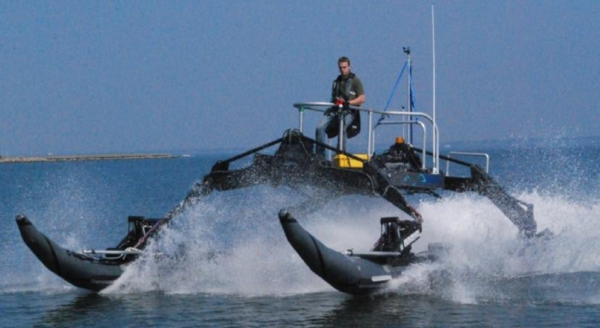Figure 2. Spray generated by the WAM-V hull at 21 knots (Peterson, 2014)

As presented above, in the developed model, the fluid data at any given time is available as discrete samples at the spatial locations occupied by the particles. At any particular instant of time, all elements of the auxiliary background mesh are labeled as water-element, air-element or interface-element. Each particle has an identity which is either air (label: ${\textstyle \,+}$$1$) or water (label: ${\textstyle \,-1}$). Within each element and for a given time-step, the particles transfer their identities to the element nodes using equations (10). After the assembly of the identities, each node has a value between ${\textstyle \,-}$$1$ and ${\textstyle \,+1}$. Then, a continuous piecewise linear approximation of the otherwise discrete identity data is obtained on the background mesh. The water-air interface is defined as the piecewise planar surface where the aforesaid approximate identity takes a value 0.

In due course, situations may arise where we will find water particles on the air-side of the interface. These particles compose the spray generated in the simulations (see Figure 3). Should the pockets of water particles be large enough then it creates a situation where there are one or more water-elements surrounded by air-elements. These islands of water-elements are seen as water splash in the simulations which represent violent separation and/or merger of the interface. Naturally, such representation of water spray and its intensity depends on the number of particles chosen in the simulation. Nevertheless, the number of particles that compose the spray is not a representation of the mass of water in the spray. Recall that particles represent material points that carry with them only the intrinsic properties of the flow. So a smaller number of particles just mean that the spray representation is sampled at a coarser level of detail.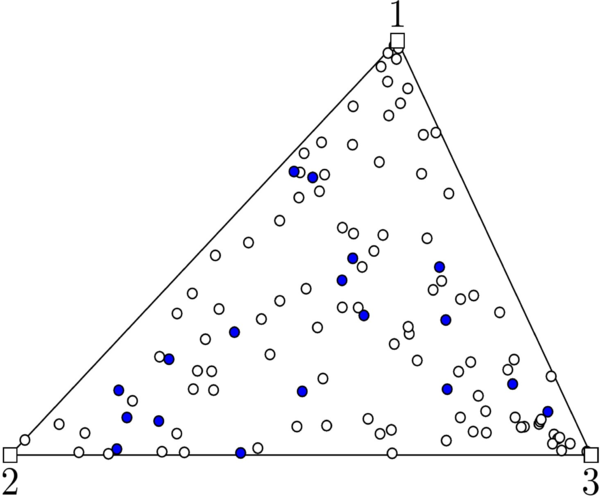Figure 3. Particles that compose the spray in an air-element. The water particles are shown as blue circles; the air particles are seen as white circles.

It is important to emphasize that other possible physical conditions which may generate spray are not considered in our model. For instance, the viscous action of air motion may separate water particles from the interface or decompose existing water splash into water spray. Additionally, this phenomenon can happen at multiple scales wherein entities which can be classified as water spray at a coarse scale may be classified as water splash at a fine scale (which in turn can be decomposed into spray). This cascade will continue until surface tension forces comes to prominence and protect the integrity of the water droplets. Reproducing such physical conditions is out of the scope of this work.

## ANALYSIS OF THE WAM-V USING THE SL-PFEM

As stated above, one of the primary concerns of this project is the simulation of the WAM-V under spray generating conditions. This section presents an application example of the SL-PFEM solver, including the modelling of the spray generation of the WAM-V craft, following the procedure presented in the previous sections. In order to reduce the computational effort required, only a symmetric configuration (with forward monochromatic seas) has been studied. Therefore, we just simulate the action of only one hull of the craft, neglecting the possible interaction of the deck. Anyhow, these simulations will allow us to obtain a qualitative understanding of the physical conditions leading to spray generation.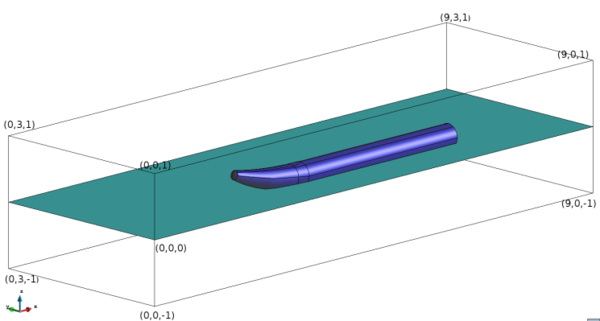Figure 4. The domain dimensions, location of the hull and the waterline

The problem domain is a 3D box with straight walls (see Figure 4). The domain dimensions are: 9 m along the x-axis, 3 m along the y-axis and 2 m along the z-axis. The coordinates (0.0,0.0,-1.0) and (9.0,3.0,1.0) represent two diagonally opposite corners of the domain. The face with coordinates (0.0,0.0,-1.0), (9.0,0.0,-1.0), (9.0,0.0,1.0) and (0.0,0.0,1.0) represents the plane of symmetry of the WAM-V. The CAD geometry of the problem domain is obtained by subtracting the volume occupied by the catamaran-type hull of the WAM-V from the volume of the containing 3D box. The geometry of the hull used in the simulations corresponds to the 14 feet (4.27 meters) USV configuration of the WAM-V. The draft of the hull, i.e. the displacement below the steady waterline is taken as 4 inches.

The domain is discretized by a mesh of 3,080,211 three-node tetrahedral elements. On an average twenty particles (material points that transport intrinsic properties of the fluid) per element were used in the CFD simulations, resulting in approximately 60 million particles.

The auxiliary background mesh is deformed every time step such that its internal boundary always conforms to that of the WAM-V hull. The mesh deformation scheme used is based on a Laplacian solver which is commonly used in the implementation of the Arbitrary Lagrangian–Eulerian formulations (Oñate et al., 2004). This enables us to impose the no-slip velocity boundary conditions at the internal boundary in a straight-forward manner.

Since the interest of this study is focused on the near field, the solution scheme used in this case assumes a flat incident free surface field. Therefore, the water wave motion is generated by imposing the solution of a first-order Stokes wave in a narrow strip of water at the inlet and on the walls of the outlet. The analytical solution of the first-order Stokes wave used here is given by

 $a=0.05\,m,\,\,k={\frac {4\pi }{3}},\,\,$ $g=9.8\,m{/s}^{2},\,\,\omega ={\sqrt {gk}}$ $u\left(x,y,t\right)==a\mathrm {exp} \,\left(kz\right)\mathrm {cos} \,\left(k\left(x-Ut\right)-\omega t\right)+$$U$ $v\left(x,y,t\right)=a\mathrm {exp} \,(kz)\mathrm {sin} \,\left(k\left(x-Ut\right)-\omega t\right)$
(15)

where ${\textstyle a}$ is the amplitude of the wave, ${\textstyle k}$ is the angular wavenumber, ${\textstyle g}$ is the acceleration due to gravity and ${\textstyle \omega }$ is the angular frequency. Further U is the velocity with which the WAM-V moves relative to water and ${\textstyle u\left(x,y,t\right),\,v(x,y,t)}$ represent the spatial velocity components of the water in an inertial reference frame that moves with the WAM-V. The narrow strip at the inlet where the wave velocity is imposed has a width of 0.2m. This periodic velocity condition causes a disturbance which is propagated in the rest of the domain and whose motion is governed by the Navier-Stokes equations. In this case, only the near field of the problem is analyzed, and therefore the standard formulation of the fluid dynamics solver (i.e. not the diffraction-radiation splitting formulation) has been used.

The total physical time of simulation was chosen as 4s. The time evolution was computed using 800 time steps of 0.005s each.

The results of three representative cases are here presented. These correspond to three different speeds of the WAM-V, viz. U=15 knots, U=20 knots and U=25 knots, respectively.

Figure 5. Simulation of the spray generated by the 14 ft WAM-V hull at 25 knots using the PFEM/X-IVAS method.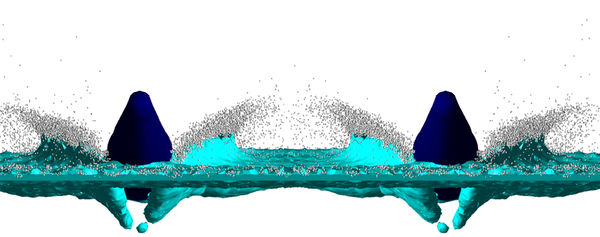Figure 6. Details of the spray generated by the 14 ft WAM-V hull at 15 knots; YZ plane; Time = 3.66s.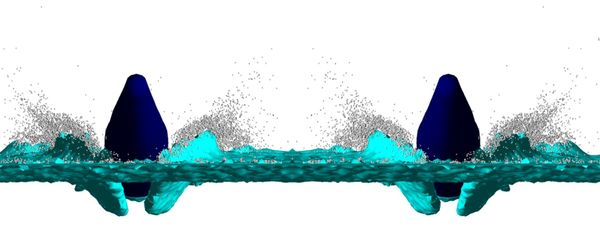Figure 7. Details of the spray generated by the 14 ft WAM-V hull at 20 knots; YZ plane; Time = 3.59s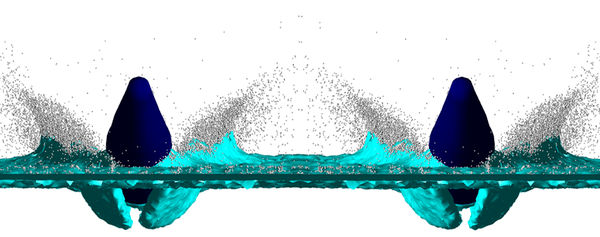Figure 8. Details of the spray generated by the 14 ft WAM-V hull at 25 knots; YZ plane; Time = 3.52s

Next, a video of the spray generated by the 14 ft WAM-V hull at 25 knots is presented.

 Video 1. Spray generated by the 14 ft WAM-V hull at 25 knots.

As discussed in the previous section, the blue isosurface drawn in the pictures corresponds to the piecewise planar surface where the projected identity of the particles on the nodes takes a value 0. It has to be emphasized that this projection process implies a relevant loss of resolution; the flow is actually solved with twenty particles per element (on average) but the blue surface is constructed only with the weighted values on the nodes of the linear elements. In the zones where the developed flow involves a complex mixture of air and water, the free surface cannot be identified. In that case, the calculated isosurface should be understood only as a reference; below that isosurface we will find water with a relatively small proportion of air bubbles, and above it, we will find an increasing presence of air. In particular, the isosurface shows big bulges below the hull that should not be understood as air pockets, but as volumes where the air-water mixing phenomenon is quite complex.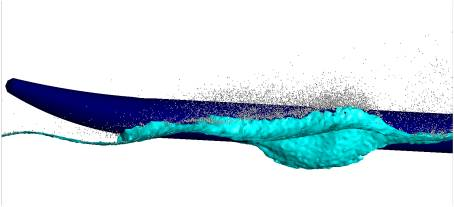Figure 9. XY plane view of the spray generated by the 14 ft WAM-V hull at 25 knots and time = 3:57 s.

All the run cases took nearly 24 hours each to perform the computations using a workstation with an Intel®CoreTMi7 - 3820 CPU and 32 GB RAM. The computations were performed using the four (available) cores thanks to the parallelization capabilities of the implemented solver. The memory requirements of these simulations were nearly 17 GB of RAM.

## CONCLUSIONS

In the different simulations of the WAM-V, we have seen that the semi-Lagrangian Particle Finite Element method can a suitable tool for the analysis of incompressible flows subjected to challenging physical conditions, e.g. violent interface motions, spray generating conditions, etc.

Due to the Lagrangian treatment of the advective processes and the Lagrangian data storage strategy in the SL-PFEM, the interfaces are accurately tracked.

Further, the computational task associated to advective transport is mutually exclusive and hence scalable on parallel computers.

The paper has presented a proposal to represent the generation of water spray based on the discrepancies between the intrinsic information stored in the particles (which is either air or water) and the projected information into the mesh nodes. Nevertheless, the number of particles that compose the spray in the calculation is not a representation of the mass of water in the spray. However, this result can be an indicator of the intensity of the generated spray, which could be used to design and evaluate strategies to reduce the spray generation of the vessel.

Reproducing the complex physics of the different phenomena involved in the spray generation was out of the scope of this work.

## ACKNOWLEDGEMENTS

This study was partially supported by the WAM-V project funded under the Navy Grant N62909-12-1-7101 issued by Office of Naval Research Global, the SAFECON project (ref. 267521, FP7-IDEAS-ERC), the FORECAST project (ref. 664910, H2020-ERC-2014-PoC) and the X-SHEAKS project (ref. ENE2014-59194-C2-1-R). The United States Government has a royalty-free license throughout the world in all copyrightable material contained herein.

Permission to use the image shown in Figure 2 has been granted by Prof. Mehdi Ahmadian, VirginiaTech, USA. This image has appeared earlier in Andrew William Peterson’s Ph.D. thesis (2014), figure 3.12, page 55.

Back to Top

### Document informationPublished on 22/12/16

Licence: CC BY-NC-SA license

### Document Score5

Views 143
Recommendations 1

### Keywords### claim authorship

Are you one of the authors of this document?